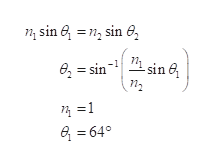# A light beam containing red and violet wavelengths is incidenton a slab of quartz at an angle of incidence of 50.00°. Theindex of refraction of quartz is 1.455 at 660 nm (red light),and its index of refraction is 1.468 at 410 nm (violet light).Find the dispersion of the slab, which is defined as the differencein the angles of refraction for the two wavelengths.

Question
1 views

A light beam containing red and violet wavelengths is incident
on a slab of quartz at an angle of incidence of 50.00°. The
index of refraction of quartz is 1.455 at 660 nm (red light),
and its index of refraction is 1.468 at 410 nm (violet light).
Find the dispersion of the slab, which is defined as the difference
in the angles of refraction for the two wavelengths.

check_circle

Step 1

Now use Snell’s law for this problem,help_outlineImage Transcriptionclose1, sin 6 =n, sin e, e, = sin- 1 sin 6, =1 e = 64° fullscreen
Step 2

Now for red light n2=1.455

Then,

Step 3

For violet light n2=...

### Want to see the full answer?

See Solution

#### Want to see this answer and more?

Solutions are written by subject experts who are available 24/7. Questions are typically answered within 1 hour.*

See Solution
*Response times may vary by subject and question.
Tagged in

### Ray Optics